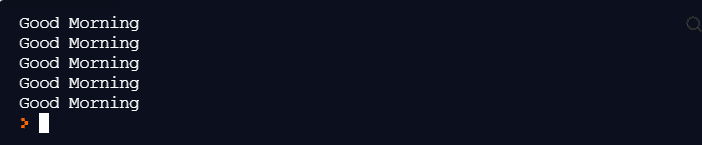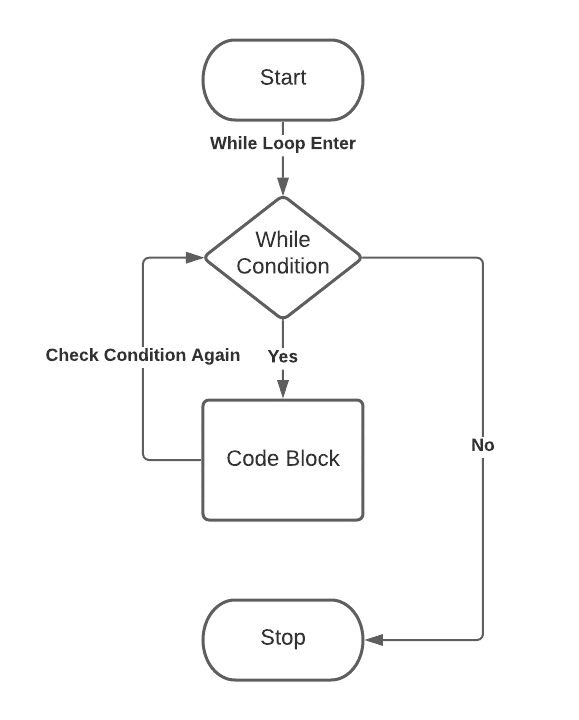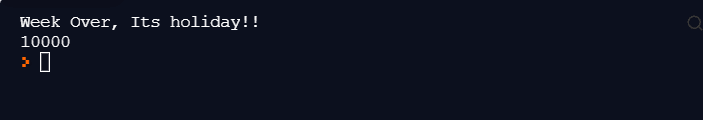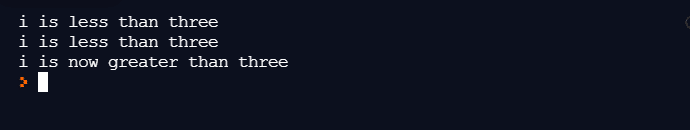In the last post about Python "for" loops, we got introduced to Python's concept and how does Python handle loop. With different variations and methods introduced in loops, we are all set to move ahead to the next and probably the only other important loop in Python: python while loop. Since this is also a loop, the work needs no introduction in this post. If you are unaware, I highly recommend going through the python "for" loops and brief yourselves with the basics.

This post will cover the basics in the following fields:

• What is the Python "While" loop?
• Syntax of Python "while" loop.
• How to implement while loops in Python?
• Flowchart for Python While loops.
• While True in Python
• While-Else in Python
• Python "Do While" loops.

## What is the Python "While" loop?

The while loop in python is a way to run a code block until the condition returns true repeatedly. Unlike the "for" loop in python, the while loop does not initialize or increment the variable value automatically. As a programmer, you have to write this explicitly, such as "i = i + 2". It is necessary to be extra cautious while writing the python "while" loop as these missing statements can lead to an infinite loop in python. For example, if you forgot to increment the value of the variable "i" , the condition "i < x" inside "while" will always return "True". It is therefore advisable to construct this loop carefully and give it a read after writing.

### The syntax for Python while loops

The syntax for python while loop is more straightforward than its sister "for" loop. The while loop contains only condition construct and the piece of indented code, which will run repeatedly.

``````while(condition):
//Code Block
``````

The conditions can be as simple as (i < 5) or a combination of them with the boolean operators' help in python. We shall see them steadily into the post.

### How to implement a while loop in Python?

To implement the while loop in Python, we first need to declare a variable in our code as follows (since initialization is not like the for loop):

``````i = 1
``````

Now I want "Good Morning" to be printed 5 times. Therefore, the condition block will look as follows:

i <= 5

The indented code will be the code I would like to execute when the condition returns True.

``````print("Good Morning")
i = i + 1
``````

Combining my code, it will look as follows:

``````i = 1
while(i <= 5):
print("Good Morning")
i = i + 1
``````

When we compile and run the code, the following iterations occur during the loop execution:

Iteration 1: i = 1; i <= 5 return True; Good Morning printed

Iteration 2: i = 2; i <= 5 return True; Good Morning printed

....

Iteration 5: i = 5; i <=5 return True; Good Morning printed

Iteration 6: i = 6; i <= 5 returns False; Loop ExitCan you guess what would happen if I skip the line i = i + 1? Run it and find out!

If the code block inside the while loop is a single statement, the programmer can also write the while loop. Moreover, the statement in a single line as follows:

``````while(condition):   Single Statement
``````

### Flowchart for Python While loops

Now that we know the working and construction of the while loop, we can visualize the loop's flow through a flowchart. The flowchart of python "while" loop would look as follows:I hope it comes in handy while practicing for the “while” loop in python.

### While True in Python

There is a concept of declaring a condition true in python or any other programming language without evaluating any expression. It is a practice followed to indicate that the loop has to run until it breaks. We then write the break statements inside the code block.

The while true in python is simple to implement. Instead of declaring any variable, applying conditions, and then incrementing them, write true inside the conditional brackets.

``````while(True):
//code block
``````

The following code will run infinitely because "True" is always "True" (no pun intended!).

``````while(True):
print("Good Morning")

``````

Use this condition carefully as if your break statement is never touched. Your loop will continuously eat the resources and waste time. Typically, while true in python is used with a nested if-else block, but this is not standard, and there is no such rule. Every program has its demands, and as you work your way forward, you will be able to implement this with variations. I have shown a program to implement in the snippet below while true in python with a sure path of exit.

``````weekSalary = 0
dayOfWeek = 1
week = ["Monday", "Tuesday", "Wednesday", "Thursday", "Friday", "Saturday", "Sunday"]
while(True):
if(week[dayOfWeek] == "Sunday"):
print("Week Over, Its holiday!!")
break
weekSalary += 2000
dayOfWeek += 1

print(str(weekSalary))
``````

Can you think out the output of this code using the iteration process?The example above uses python lists and breaks statements in the code. Please refer to python lists and break statements in python to learn more about them.

### While-Else in Python

The while loop in python also supports another interesting use case. If there are any program requirements to execute a sentence after the loop, we can construct an "else" statement that would execute when the condition returns "False." After the "else" statement, the loop exits.

``````while(condition):
//code block
else:
//code block
``````

A small example to demonstrate the same is as below:

``````i = 2
while(i <= 3):
print("i is less than three")
i = i + 1
else:
print("i is now greater than three")
``````

The above code prints the following output onto the console:Alright! A good question to think here is, what happens when we have a break statement along with the else statement? Do else statements get skipped? Or does "else" get executed?

If there is a while loop in python with a break statement and an else statement, the else statement skips when the"break" executes. It would be a good exercise to run a while loop in Python with a break and else statement.

You can also implement the for-else statement in the for loop, and it works exactly as same as here. The following syntax will help you build your for-else loop in the code:

``````for(conditions):
//code
else:
//code
``````

### Python "do while" loop

In case you are coming from another programming language such as C++, you might have used a "do while" loop and would be interested in knowing how to implement the same in python. Unfortunately, for "do while" fans, this loop is not supported by python. I feel the "do while" loop is redundant as it is similar to the "while" loop except for the first iteration, which is bound to run necessarily in "do-while."

Leaving the standard textbook facts aside, can you try to build a do-while loop in python by yourself? The "do-while" loop always executes the first iteration and then checks the condition to decide if another iteration would run or not. To understand it using another loop, let's dissect this statement and construct a "do-while" loop ourselves.

Statement 1:  The "do-while" loop always executes the first iteration.

This statement tells us that we do not need to check the condition while entering the code block. Does that ring any bells? Yes!!, we need a "while true" statement since it is always true. So we start with the following statement:

``````while (True):
``````

Statement 2: After that, check the condition to decide if another iteration would run or not.

Since we need to check the condition after the code block has executed once, we can put a simple if statement with a break as follows:

``````while(True):
//Code Blocks
if(condition):
break
``````

The above code works exactly similar to the following do-while loop:

``````do {

//code block

} while(condition);
``````

It brings us to the end of this post. I hope we addressed all your queries in this post. If some doubt remains, you can raise them in the FAQ section or email me at [email protected]. I would be happy to help you out!

## Key Takeaways

• The while loop in python runs until the "while" condition is satisfied.
• The "while true" loop in python runs without any conditions until the break statement executes inside the loop.
• To run a statement if a python while loop fails, the programmer can implement a python "while" with else loop.
• Python does not support the "do while" loop. Although we can implement this loop using other loops easily.ReviewersLakshay Sharma